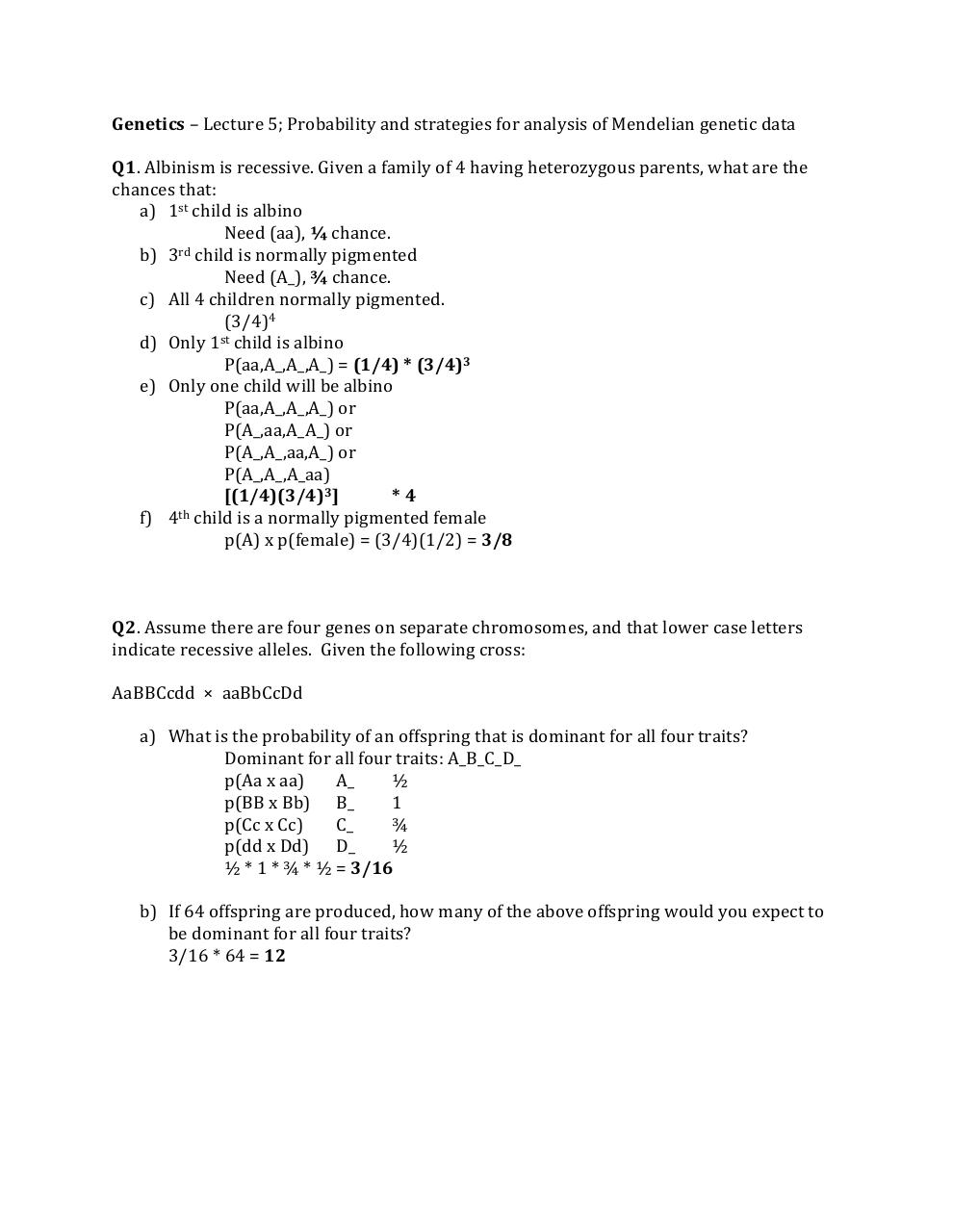PDF Archive

Easily share your PDF documents with your contacts, on the Web and Social Networks.

Notes Lecture 5; Strategies for analysis.pdfPage 1 2 3

Text preview

Genetics – Lecture 5; Probability and strategies for analysis of Mendelian genetic data
Q1. Albinism is recessive. Given a family of 4 having heterozygous parents, what are the
chances that:
a) 1st child is albino
Need (aa), ¼ chance.
b) 3rd child is normally pigmented
Need (A_), ¾ chance.
c) All 4 children normally pigmented.
(3/4)4
d) Only 1st child is albino
P(aa,A_,A_,A_) = (1/4) * (3/4)3
e) Only one child will be albino
P(aa,A_,A_,A_) or
P(A_,aa,A_A_) or
P(A_,A_,aa,A_) or
P(A_,A_,A_aa)
[(1/4)(3/4)3]
*4
th
f) 4 child is a normally pigmented female
p(A) x p(female) = (3/4)(1/2) = 3/8

Q2. Assume there are four genes on separate chromosomes, and that lower case letters
indicate recessive alleles. Given the following cross:
AaBBCcdd × aaBbCcDd
a) What is the probability of an offspring that is dominant for all four traits?
Dominant for all four traits: A_B_C_D_
p(Aa x aa)
A_
½
p(BB x Bb) B_
1
p(Cc x Cc)
C_
¾
p(dd x Dd) D_
½
½ * 1 * ¾ * ½ = 3/16
b) If 64 offspring are produced, how many of the above offspring would you expect to
be dominant for all four traits?
3/16 * 64 = 12